#### Click Here to Download Free E-books

Physical Properties of Polymers | Textile Study Center
Home Wet Processing Eng. Man-Made Fiber Physical Properties of Polymers

# Physical Properties of Polymers

2.44K
0#### Arjya Shrestho Dey## Physical Properties of Polymers

Polymer:

A chemical compound or mixture of compounds formed by polymerization and consisting essentially of repeating structural units is called ‘Polymer’. Polymers range from familiar synthetic plastic such as polystyrene to natural bio-polymers such as DNA and proteins that are fundamental to biological structure and function.

Physical Properties:

Physical properties of polymers include molecular weight, molar volume, density, degree of polymerization, crystallinity of material, and so on. Some of these are discussed herewith in the following sections.

Degree of Polymerization and Molecular Weight:

First of all, let us discuss the degree of polymerization. The degree of polymerization (DP)-n in a polymer molecule is defined as the number of repeating units in the polymer chain. For example,

−(−CHCH −)−n

The molecular weight of a polymer molecule is the product of the degree of polymer-ization and the molecular weight of the repeating unit. The polymer molecules are not identical but are a mixture of many species with different degrees of polymerization, that is, with different molecular weights. Therefore, in the case of polymers we talk about the average values of molecular weights.

Molecular Weight Averages:

Suppose we have a set of values {x1, x2, … , xn} and the corresponding probability of occurrence is given by {P1, P2, … , Pn}, then the average value is defined as follows: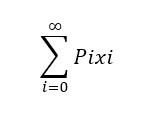Number-Average Molecular Weight:

If Ni is the number of polymer molecules having the molecular weight Mi, then the “number-average” probability of the given mass is given below: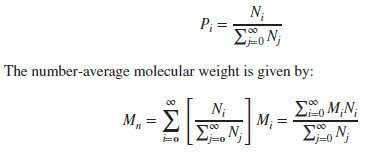The physical properties (such as transition temperature, viscosity, etc.) and mechanical properties (such as strength, stiffness, and toughness) depend on  molecular weight of polymer. The lower the molecular weight, lower the transition temperature, viscosity, and the mechanical properties. Due to increased entanglement of chains with increased molecular weight, the polymer gets higher viscosity in molten state, which makes the processing of polymer difficult.

Weight-Average Molecular Weight:

The weight-average prob-ability is given by: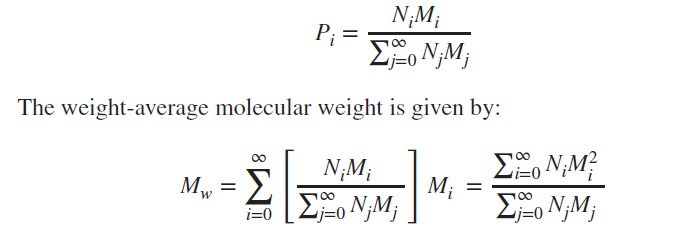A typical plot showing the number-average and weight-average molecular weight is shown in following figure. The number-average molecular weight is less than the weight-average molecular weight The degree of polymerization can be calculated using the number-average molecular weight.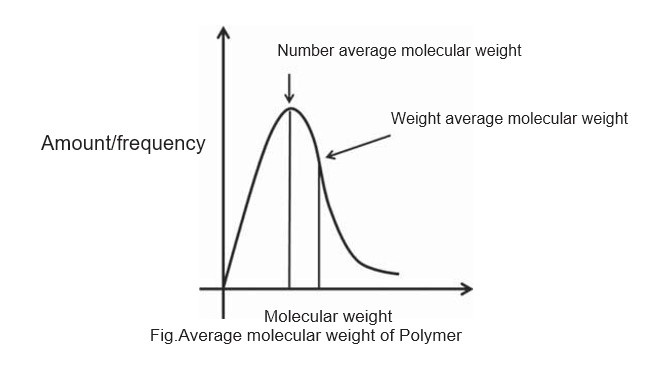Polydispersity Index or Heterogeneity Index:

The ratio of the weight-average molecular weights to the number-average molecular weights is called polydispersity index (PDI) or heterogeneity index, which measures the polydispersity of the polymer mixture.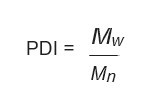The dispersity measures heterogeneity of sizes of molecules or particles in the mix-ture. The mixture is called monodisperse if the molecules have the same size, shape, or mass. If the molecules in the mixture have an inconsistent size, shape and mass distri-bution, the mixture is called polydisperse.

The natural polymers are generally monodisperse as all synthetic polymers are poly-disperse with some exceptions. The PDI is equal to or greater than 1 where as the polymer chains approach uniform chain length, the PDI tends to unity.

## Crystallinity:

The state where the polymer chains exist in parallel position that a polymer can be achieve at a particular temperature. It depends on chemical nature, geometrical structure, molecular weight and molecular weight distribution etc. Maximum crystallinity become possible if the polyer is annealed just at its Tm  (Melting Temperature) for sufficiently long time.

Crystallinity is expressed as degree of crystallinity, given by,Where,

C     = Refractive index/density of whole polymer

Cam = The value of property when the polymer is amorphous.

Ccr   = The value of property when the polymer is crystalline.

Crystallinity increases with density.

## Amorphous:

The state of polymer where the chains are palced in haphazard direction is termed as  the amorphousness of polymer. The density of polymer decreases of this area.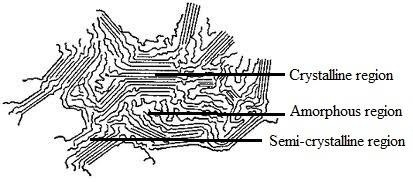## Semi-crystalline:

The state between crystalline and amorphous of a polymer is called semi crystalline.

## Comparison of properties of crystalline and amorphous polymer:

 Properties Crystalline Amorphous 1. Strength High Less 2. Melting point high Less 3. Elongation Less high 4. Dye takeup Less high 5. Light deflection Regular Irregular 6. Density high Less 7.Phase Crystalline Liquid 8. Structure Linear Branch or random 9. Polarity high Less 10. Flexural modulus high Less 11. Hardness high Less 12. Permeability Less high 13. Acid hydrolysis Difficult Attack rapidly 14. Young modulus high Less 15. Chain mobolity easy high

Factor that Control Crystallinity of Polymer:

1. Regularity of the molecular structure of the polymer chain: In general, crystalline substance is of regular molecular
2. Polarity: Polarity increases the crystallinty of a polymer
3. The mobility of polymer chain: The mobility of polymer chain is another factor that control crystallinity of polymer. If the polymer chain is mobile, the polymer will be

## Extended chain crystals:

1. Less common, often take a needle
2. Usually formed with low molecular weight polymer by slow crystallization or under pressure.

Nucleation – onset of crystallinity.

1. Homogeneous nucleation – occur randomly throughout the
2. Heterogeneous nucleation –occur at the interface of a foreign impurity 9e.g. a finely divided silica).

(2439)#### Arjya Shrestho Dey### Comments

comments

Powered by Facebook Comments

Close